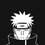# General sum

Can anyone tell me how to calculate general sum of sequence till $n$ . $1^{4}+2^{4}+3^{4}+ \ldots +n^{4}$Note by Akshat Sharda
5 years, 9 months ago

This discussion board is a place to discuss our Daily Challenges and the math and science related to those challenges. Explanations are more than just a solution — they should explain the steps and thinking strategies that you used to obtain the solution. Comments should further the discussion of math and science.

When posting on Brilliant:

• Use the emojis to react to an explanation, whether you're congratulating a job well done , or just really confused .
• Ask specific questions about the challenge or the steps in somebody's explanation. Well-posed questions can add a lot to the discussion, but posting "I don't understand!" doesn't help anyone.
• Try to contribute something new to the discussion, whether it is an extension, generalization or other idea related to the challenge.
• Stay on topic — we're all here to learn more about math and science, not to hear about your favorite get-rich-quick scheme or current world events.

MarkdownAppears as
*italics* or _italics_ italics
**bold** or __bold__ bold
- bulleted- list
• bulleted
• list
1. numbered2. list
1. numbered
2. list
Note: you must add a full line of space before and after lists for them to show up correctly
paragraph 1paragraph 2

paragraph 1

paragraph 2

[example link](https://brilliant.org)example link
> This is a quote
This is a quote
    # I indented these lines
# 4 spaces, and now they show
# up as a code block.

print "hello world"
# I indented these lines
# 4 spaces, and now they show
# up as a code block.

print "hello world"
MathAppears as
Remember to wrap math in $$ ... $$ or $ ... $ to ensure proper formatting.
2 \times 3 $2 \times 3$
2^{34} $2^{34}$
a_{i-1} $a_{i-1}$
\frac{2}{3} $\frac{2}{3}$
\sqrt{2} $\sqrt{2}$
\sum_{i=1}^3 $\sum_{i=1}^3$
\sin \theta $\sin \theta$
\boxed{123} $\boxed{123}$

## Comments

Sort by:

Top Newest

Study Faulhaber's Formula for a General Idea. Also visit Bernoulli's numbers if it interests you. :)

- 5 years, 9 months ago

Log in to reply

1/30(n+1)(2n+1)(3n^2+3n-1)

- 5 years, 9 months ago

Log in to reply

Hint:

\begin{aligned} \sum_{k=1}^n \left( (k+1)^2 - k^2 \right) &= &2 \sum_{k=1}^n k + \sum_{k=1}^n 1 \\ \displaystyle (n+1)^2 - 1 &=& 2 \color{#3D99F6}{\sum_{k=1}^n k} + n \\ \displaystyle \sum_{k=1}^n k &=& \frac{n(n+1)}2 \end{aligned}

And

\begin{aligned} \sum_{k=1}^n \left( (k+1)^3 - k^3 \right) &= & \sum_{k=1}^n (3k^2 + 3k + 1) \\ \displaystyle (n+1)^3 - 1 &=& 3\color{#3D99F6}{ \sum_{k=1}^n k^2} +3 \sum_{k=1}^n k + \sum_{k=1}^n 1 \\ \displaystyle (n+1)^3 - 1 &=& 3\color{#3D99F6}{ \sum_{k=1}^n k^2} +3\frac{n(n+1)}2 + n \\ \sum_{k=1}^n k^2 &=& \frac16 n(n+1)(2n+1) \\ \end{aligned}

Can you find a general pattern to it?

Answer is $\frac1{30} n(n+1)(2n+1)(3n^2 + 3n-1)$. You can double check your work by induction.

- 5 years, 9 months ago

Log in to reply

I have a question- Will this pattern work to find general sum of any power of $n$?

- 5 years, 9 months ago

Log in to reply

Study Bernoulli's Numbers. You'll get the answer for nth power sums. :)

- 5 years, 9 months ago

Log in to reply

I find that more troublesome, unless you got a table for the number of Bernoulli numbers, then yes, it will be easier, otherwise it takes up more time.

- 5 years, 9 months ago

Log in to reply

Well, it's true. I was just giving a general idea! :)

- 5 years, 9 months ago

Log in to reply

For positive integer powers, yes.

- 5 years, 9 months ago

Log in to reply

I appriciate what you did and Thank you for the same.

- 5 years, 9 months ago

Log in to reply

(6x + 15x + 10x - x) / 30

- 5 years, 9 months ago

Log in to reply

Hm, that simplifies to just $x$. Are you missing some exponents?

Staff - 5 years, 9 months ago

Log in to reply

Even I know the formula but how to derive it is much more important.

- 5 years, 9 months ago

Log in to reply

×

Problem Loading...

Note Loading...

Set Loading...